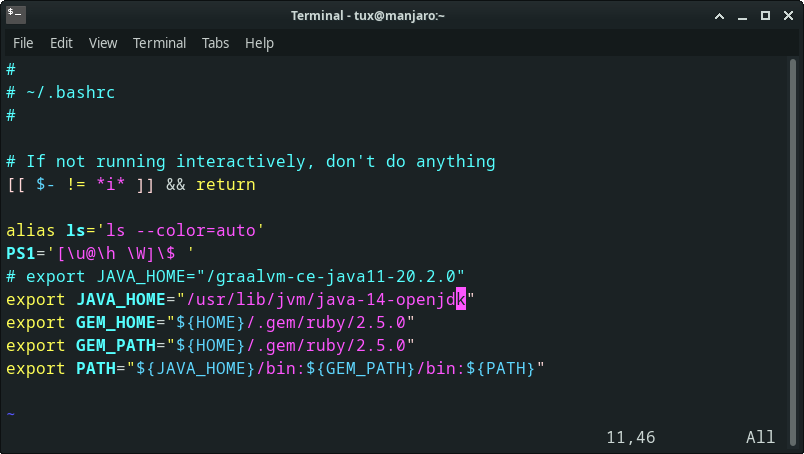Recently I’ve been working on Newton Fractal Sketches using ruby Complex Math, which are quite testing for the RaspberryPI processor. Up till now I’d been reasonably happy with the performance of OpenJDK14 with my PiCrate sketches, but the Newton Fractal sketches run quite slowly (especially compared with processing.py and jython). So finally I bit the bullet and gave the latest GraalVM a go, which is now available for aarch64. Running on Manjaro ARM OS, I installed Graal on `/opt`, I then unset archlinux-java and used my `~/.bashrc` to control which jvm was used:-Here is a test sketch

``````# frozen_string_literal: true

require 'picrate'

class NewtonFractal < Processing::App
IMGX = 512
IMGY = 512
MAXIT = 20 # max iterations allowed
EPS = 1e-3 # max error allowed
COMPLEX = Complex(1e-6, 1e-6) # step increment

attr_reader :xa, :xb, :ya, :yb, :z, :img, :start

def settings
size(IMGX, IMGY)
end

def setup
sketch_title 'Newton Fractal'
#color_mode(HSB)
@start = Time.now
# Drawing area
@xa = -1.0
@xb = 1.0
@ya = -1.0
@yb = 1.0
@img = create_image(width, height, RGB).tap do |image|
grid(IMGY, IMGX) do |y, x|
zy = y * (yb - ya) / (IMGY - 1) + ya
zx = x * (xb - xa) / (IMGX - 1) + xa
@z = Complex(zx, zy)
(0...MAXIT).each do |i|
# Newton iteration
z0 =  z - func(z) / ((func(z + COMPLEX) - func(z)) / COMPLEX)
break if (z0 - z).abs2 < EPS * EPS

@z = z0
# pixels[x + y * width] = color(i % 5 * 64, i % 17 * 16, i % 9 * 32)
image.pixels[x + y * width] = color(i % 5 * 64, i % 9 * 32, i % 17 * 16)
end
end
end
no_loop
end

def draw
set(0, 0, img)
puts Time.now - start
end

def func(z)
z**3.0 - 1.0
# z**4 - 1.0
end
end

NewtonFractal.new

``````

This sketch took over 2 minutes to develop on OpenJDK14 and less than a minute to develop on the GraalVM. Also I found a community PKGBUILD build, which require a `fakeroot`, install as follows (then you can use `archlinux-java` to switch `jvm`:-

``````sudo pacman -S fakeroot
git clone https://aur.archlinux.org/jdk11-graalvm-bin.git
cd jkd11-graalvm-bin
makepkg -si

``````